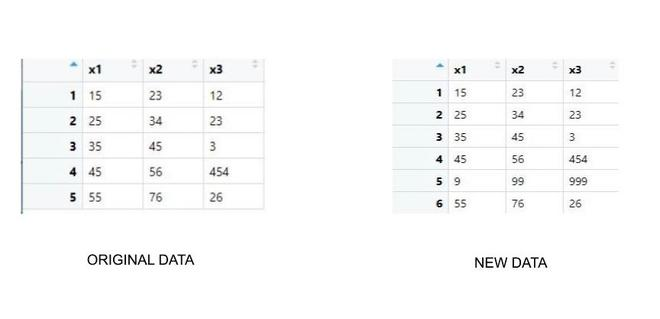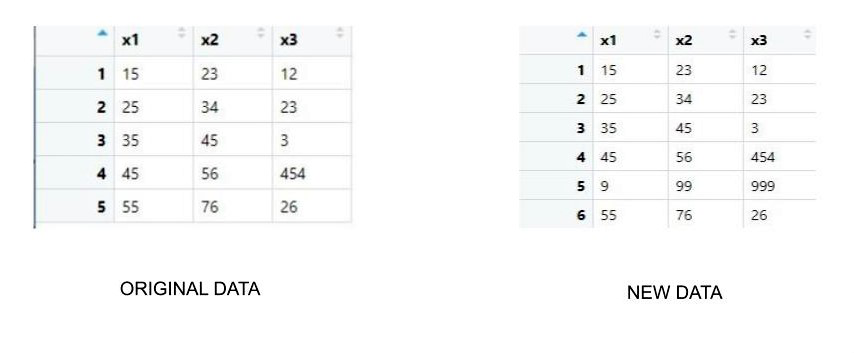Open in App
Not now

# Add New Row at Specific Index Position to Dataframe in R

• Last Updated : 21 Apr, 2021

In this article, we will discuss how to add a new row at a specific index in the dataframe in the R programming language.

The main task here is to shift other rows and make space in the dataframe for the new row and then insert its contents. This can be done in two ways

### Method 1: Using rbind()

rbind() function in R Language is used to combine specified Vector, Matrix, or Data Frame by rows.

Syntax: rbind(x1, x2, …, deparse.level = 1)

Parameters:

• x1, x2: vector, matrix, data frames
• deparse.level: This value determines how the column names generated. The default value of deparse.level is 1.

Example

## R

 `rm``(list = ``ls``())`` ` `# Function to create new dataframe``insertRow <- ``function``(data, new_row, r) {``  ``data_new <- ``rbind``(data[1:r, ],            ``                    ``new_row,                ``                    ``data[- (1:r), ])        ``  ``rownames``(data_new) <- 1:``nrow``(data_new)    ``  ``return``(data_new)``}`` ` `existingDF <- ``data.frame``(x1 = ``c``(15,25,35,45,55),    ``                         ``x2 = ``c``(23,34,45,56,76),``                         ``x3 = ``c``(12,23,3,454,26))`` ` `index <- 4                                             `` ` `newrow <- ``c``(9, 99, 999)                                ``newDF=``insertRow``(existingDF, newrow, index)`` ` `print``(newDF)`

Output:### Method 2: Using seq()

Another approach of doing the same is to use seq() function. Here the approach is the same, but the method is somewhat different. We are accessing rows of the dataframe and creating the space in it. Also, we used nrows() to count the total number of rows.

seq() function in R Language is used to create a sequence of elements in a Vector. It takes the length and difference between values as an optional argument.

Syntax: seq(from, to, by, length.out)

Parameters:

• from: Starting element of the sequence
• to: Ending element of the sequence
• by: Difference between the elements
• length.out: Maximum length of the vector

Example:

## R

 `rm``(list = ``ls``())`` ` `# Function to create new dataframe``insertRow <- ``function``(existingDF, new_row, r) {``  ``existingDF[``seq``(r+1,``nrow``(existingDF)+1),] <- existingDF[``seq``(r,``nrow``(existingDF)),] ``  ``existingDF[r,] <- new_row                         ``  ``return``(existingDF)`` ` `  ``}`` ` `existingDF <- ``data.frame``(x1 = ``c``(1,7,13,25,31),              ``                         ``x2 = ``c``(2,8,14,26,32),``                         ``x3 = ``c``(3,9,15,27,33),``                         ``x4 = ``c``(4,10,16,28,34),``                         ``x5 = ``c``(5,11,17,29,35),``                         ``x6 = ``c``(6,12,18,30,36))`` ` `r <- 4                              `` ` `new_row <- ``c``(19,20,21,22,23,24)                 ``newDF=``insertRow``(existingDF, new_row, r)      `` ` `print``(newDF)`

Output:My Personal Notes arrow_drop_up